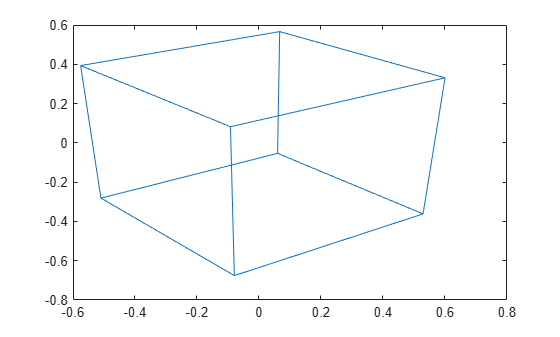Main Content

# viewmtx

View transformation matrices

## Syntax

```viewmtx T = viewmtx(az,el) T = viewmtx(az,el,phi) T = viewmtx(az,el,phi,xc) ```

## Description

`viewmtx` computes a 4-by-4 orthographic or perspective transformation matrix that projects four-dimensional homogeneous vectors onto a two-dimensional view surface (e.g., your computer screen).

`T = viewmtx(az,el) ` returns an orthographic transformation matrix corresponding to azimuth `az` and elevation `el`. `az` is the azimuth (i.e., horizontal rotation) of the viewpoint in degrees. `el` is the elevation of the viewpoint in degrees.

`T = viewmtx(az,el,phi) ` returns a perspective transformation matrix. `phi` is the perspective viewing angle in degrees. `phi` is the subtended view angle of the normalized plot cube (in degrees) and controls the amount of perspective distortion.

Phi

Description

0 degrees

Orthographic projection

10 degrees

Similar to telephoto lens

25 degrees

Similar to normal lens

60 degrees

Similar to wide-angle lens

`T = viewmtx(az,el,phi,xc) ` returns the perspective transformation matrix using `xc` as the target point within the normalized plot cube (i.e., the camera is looking at the point `xc`). `xc` is the target point that is the center of the view. You specify the point as a three-element vector, `xc = [xc,yc,zc]`, in the interval [0,1]. The default value is `xc = [0,0,0]`.

A four-dimensional homogeneous vector is formed by appending a 1 to the corresponding three-dimensional vector. For example, `[x,y,z,1]` is the four-dimensional vector corresponding to the three-dimensional point `[x,y,z]`.

## Examples

collapse all

Determine the projected two-dimensional vector corresponding to the three-dimensional point (0.5,0.0,-3.0) using the default view direction. Note that the point is a column vector.

```A = viewmtx(-37.5,30); x4d = [.5 0 -3 1]'; x2d = A*x4d; x2d = x2d(1:2)```
```x2d = 2×1 0.3967 -2.4459 ```

Create vectors that trace the edges of a unit cube.

```x = [0 1 1 0 0 0 1 1 0 0 1 1 1 1 0 0]; y = [0 0 1 1 0 0 0 1 1 0 0 0 1 1 1 1]; z = [0 0 0 0 0 1 1 1 1 1 1 0 0 1 1 0];```

Transform the points in these vectors to the screen, then plot the object.

```A = viewmtx(-37.5,30); [m,n] = size(x); x4d = [x(:),y(:),z(:),ones(m*n,1)]'; x2d = A*x4d; x2 = zeros(m,n); y2 = zeros(m,n); x2(:) = x2d(1,:); y2(:) = x2d(2,:); plot(x2,y2)```Use a perspective transformation with a 25 degree viewing angle.

```A = viewmtx(-37.5,30,25); x4d = [.5 0 -3 1]'; x2d = A*x4d; x2d = x2d(1:2)/x2d(4)```
```x2d = 2×1 0.1777 -1.8858 ```

Transform the cube vectors to the screen and plot the object.

```A = viewmtx(-37.5,30,25); [m,n] = size(x); x4d = [x(:),y(:),z(:),ones(m*n,1)]'; x2d = A*x4d; x2 = zeros(m,n); y2 = zeros(m,n); x2(:) = x2d(1,:)./x2d(4,:); y2(:) = x2d(2,:)./x2d(4,:); plot(x2,y2)```## See Also

### Topics

Introduced before R2006a

Download ebook